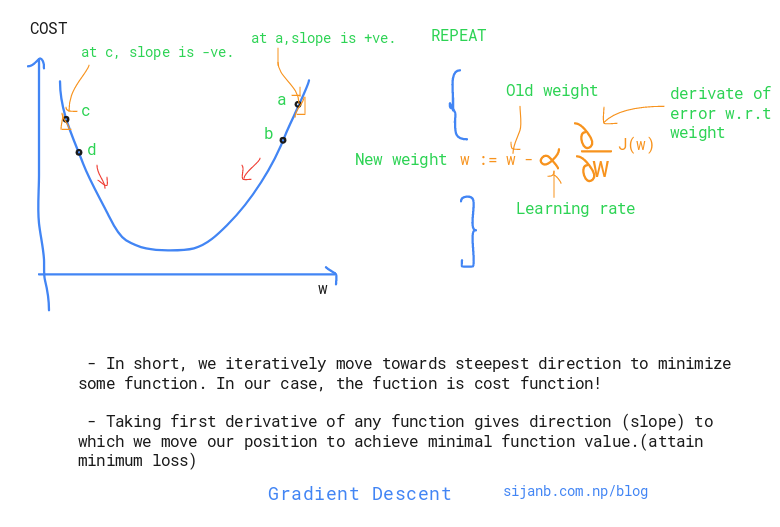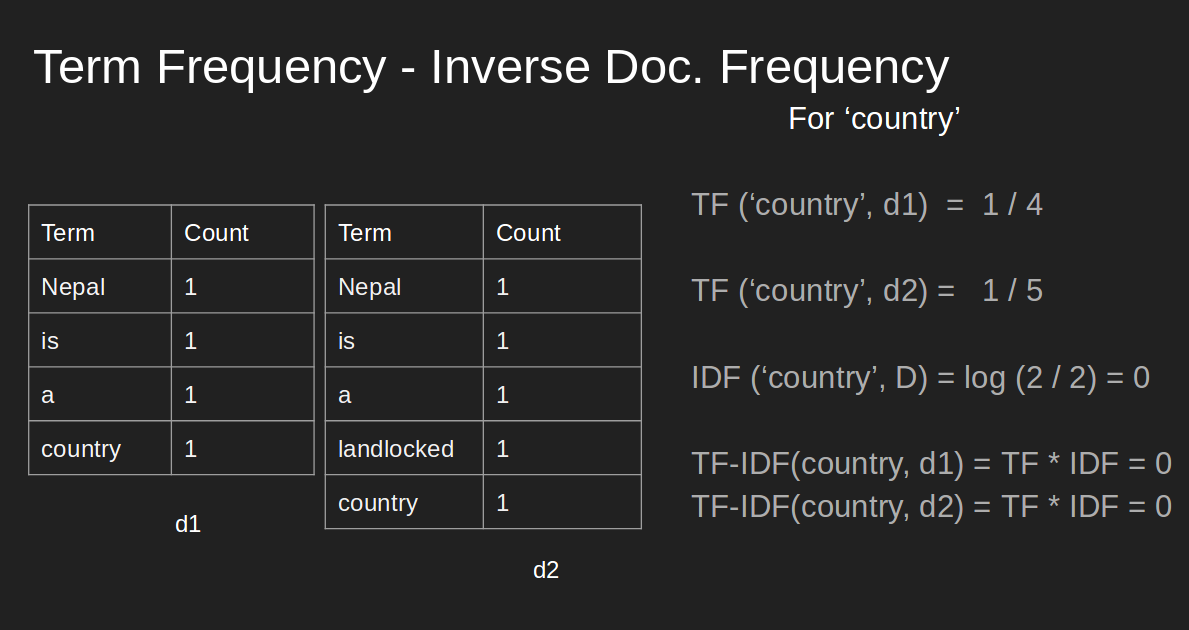Before jumping to Gradient Descent, let's be clear about difference between backpropagation and gradient descent. Comparing things make it easier to learn !

##### Backpropagation :¶

Backpropagation is an efficient way of calculating gradients using chain rule.

Gradient Descent is an optimization algorithm which is used in different machine learning algorithms to find parameters/combination of parameters which mimimizes the loss function.

** In case of neural network, we use backpropagation to calculate the gradient of loss function w.r.t to weights. Weights are the parameters of neural network.

** In case of linear regression, coefficients are the parameters!.

** Many machine learning algorithms are convex problems, so using gradient descent to get extrema makes more sense. For example, if you remember the solution of linear regression :

$\beta = (X^T X)^{-1} X^T y$

Here, we can get the analytical solution by simply solving above equations. But, inverse calculation has $O(N^3)$ complexity. It will be worst if our data size increases.

Sijan Bhandari on

Network has been taken as a tool for describing complex systems or interactions around us. Few prominent complex systems are:

1. Our society where almost 7 billions individuals exist/ and the interactions between them in one or other ways.

2. Genes in our body, interactions between gene molecules ( Protein-Protein interaction networks)

Peoply usually visualize the network to see cluter/ densely linked clusters and try to analyze, predict relation between nodes, figure out similarity between nodes in the network.

Figuring out the central nodes/vertices is also an important network analysis process because centrality measures :

        a. Existing influence of a node on other nodes
b. Information flow in and out from a node or towards it
c. Finding node/s which is/are acting as bridge between two different/big groups
Sijan Bhandari on

In any document, the frequency of occurrence of terms is taken as an important measure of score for that document (Term Frequency). For example : a document has total 100 words, and 30 words are 'mountains', we ,without hesitation, say that this document is talking about 'Mountains'.

But, if we only include most frequent word as our score metric, we will eventually loose the actual relevancy score of the document. Since same word could exist in number of documents and it's just frequent occurrence without adding much meaning to current context. In the above example : Suppose, there are two documents talking about 'Mt. Everest'. We obviously know that there will be higher occurrence of word 'Mountains'. But, if we use 'Term Frequecy (tf)' alone, term 'Mountains' will get highest weight rather than term 'Everest'. It's not fair. And, Inverse-Document-Frequency will tackle it.

#### Term Frequency (TF) / Normalized Term Frequency (nTF):¶

It simply measures the frequency/occurent of a term in a document. So, it gives equal important to all terms. Longer document could have large number of terms than smaller documents, so better to normalize this metric by dividing with total number of terms in the document. We also

#### Applications:¶

1. Summarizing a document by extracting keywords.
2. Comparing two documents (similary/ relevancy check)
3. Search query to documents matching for building query results for search engine
4. Weighting 'terms' in the document.

#### Inverse Document Frequency (IDF):¶

It gives the importance to more relevant/significant term in the document. It tries to lower the weights to terms having less importance. And, rare terms will get significant weights.

#### TF-IDF:¶

It tries to prioritize the terms based on their occurrence and uniqueness.

Suppose, I have two documents in my corpus and I want to give tf-idf weighting to the terms.

##### Document II : 'Nepal is a landlocked Country'¶We can see that, although, term 'country' has prominent occurrence, 'tf-idf' gives priority to word 'landlocked' and it carries more information about the document.

#### NOTE 1 :¶

These weights are eventually used for vector-space model, where each term represents the axes, and document are the vectors on that space. Since 'tf-idf' value is zero (as shown above)' this representation is very sparse.

#### NOTE 2 :¶

Suppose, we are building a search engine system. The query is also converted into vector in vector-space model and compare with documents (NOTE 1) to get the similarity between them.

In [ ]:


Sijan Bhandari on